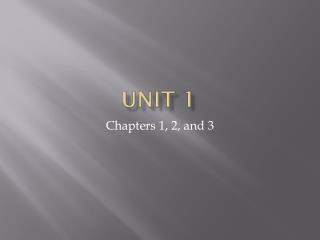# Unit 1 - PowerPoint PPT PresentationDownload PresentationUnit 1

Unit 1Download Presentation## Unit 1

- - - - - - - - - - - - - - - - - - - - - - - - - - - E N D - - - - - - - - - - - - - - - - - - - - - - - - - - -
##### Presentation Transcript

1. Unit 1 Chapters 1, 2, and 3

2. What Is Chemistry? • Chemistry is the study of matter and how it changes. • Elements: • Atoms: • Molecules and Compounds:

3. States of Matter • Solid: • Liquid: • Gas:

4. Matter

5. Pure Substnaces • Pure substance: • Mixture: • Heterogeneous: • Homogeneous:

6. Properties of Matter • Physical Properties: • Chemical Properties:

7. Intensive Properties: • Extensive Properties:

8. Atomic Theory • Subatomic Particles: • Electrostatic Forces:

10. The Nuclear Atom • Nucleus: • Electronic Charge: • Atomic Mass:

11. Atomic Size:

12. Isotopes • Isotope: • Average Atomic Mass:

13. Naturally occurring chlorine is 75.78 % 35Cl, which has an atomic mass of 34.969 amu, and 24.22 % 37Cl, which has an atomicmass of 36.966 amu. Calculate the average atomic mass of chlorine.

14. The Periodic Table • How it’s arranged:

15. Law of Definite Proportions

16. The Law of Multiple Proportions

17. Percent Mass

18. Hydrates

19. The Mole

20. Molar Mass

21. Empirical And Molecular Formula

22. Ions and Ionic Compounds • Ion: • Cation: • Anion:

23. Common Ions and their charges • Group 1: H+, Li+, Na+ • Group 2: Be2+, Mg2+, Ca2+ • Group 15: N3-, P3-, As3- • Group 16: O2-, S2-, Se2- • Group 17: F-, Cl-, Br- • Transition elements can usually have more than one charge. Example Fe2+ and Fe3+

24. Transition Metal Ions • Most transition metals can have a few different charges. • Example: • Fe2+, Fe3+ • Fe2O3 • FeO

25. Polyatomic Ions • Ammonium: NH4+ • Nitrite: NO2- • Nitrate: NO3- • Hydroxide: OH- • Hypochlorite: ClO-

26. Chlorite: ClO2- • Chlorate: ClO3- • Perchlorate: ClO4- • Carbonate: CO32- • Sulfite: SO32- • Sulfate: SO42- • Peroxide: O22- • Phosphate: PO43-

27. Naming Ionic Compounds

28. Practice • NH4Cl • Fe(NO3)3 • TiBr3 • Pb(SO4)2 • Chromium (VI) Phosphate • Tin (II) Nitrate • Cobalt (III) Oxide • Chromium (III) Hydroxide

29. Barium Carbonate • Aluminum Hydroxide • Copper (I) Sulfide • Lead (II) Phosphate • Zinc (II) Iodide • Chromium (III) Sulfite • NH4Br • NaClO3 • Fe2S3 • AgNO3 • CuF2 • Ni(ClO)3 • Ru3(PO4)4

30. Naming Molecular Compounds

31. Practice

32. Chemical Reactions • Chemical Equations:

33. Stoichiometry • How many grams of water are produced in the combustion of 1.00 g of glucose? C6H12O6(s) + 6 O2(g)  6 CO2(g) + 6 H2O(l)

34. Limiting Reactants

35. Consider the reaction that occurs in a fuel cell: 2 H2(g) + O2(g)  2 H2O(g) Suppose the fuel cell is set up with 150 g of hydrogen and 1500 g of oxygen. How many grams of water can be formed?

36. Theoretical Yield and Percent Yield

37. Adipic acid (H2C6H8O4) is used to produce nylon. The acid is made commercially by a controlled reaction between cyclohexane (C6H12) and O2: 6 C6H12(l) + 5 O2(g)  2 H2C6H8O4(l) + 2 H2O(g) a.) Assume that you carry out this reaction starting with 25.0 g of cyclohexane and that cyclohexane is the limiting reactant. What is the theoretical yeild of adipic acid? b.) If you obtain 33.5 g of adipic acid from your reaction, what is the percent yeild?

38. Example AP Questions • Complete combustion of a sample of a hydrocarbon in excess oxygen produces equimolar quantities of carbon dioxide and water. Which of the following could be the molecular formula of the compound? • C2H2 • C2H6 • C4H8 • C6H6

39. The mass percent of carbon in pure glucose, C6H12O6, is 40.0%. A chemist analyzes an impure sample of glucose and determines that the mass percent of carbon is 38.2 %. Which of the following impurities could account for the low mass percent of carbon in the sample? • Water, H2O • Ribose, C5H10O5 • Fructose, C6H12O6. An isomer of glucose • Sucrose, C12H22O11

40. How many protons, neutrons, and electrons are in a 5626Fe atom? Protons Neutrons Electrons • 26 30 26 • 26 56 26 • 30 26 30 • 56 26 26 • 56 82 56

41. Atoms of Mg combine with atoms of F to from a compound. Atoms of which of the following elements combine with atoms of F in the same ratio? • Li • Ba • Al • Cl • Ne

42. C3H8(g) + 5 O2(g)  3 CO3(g) + 4 H2O(l) • In the reaction represented above, what is the total number of moles of reactants consumed when 1.00 mole of CO2(g) is produced? • 0.33 mol • 1.33 mol • 1.50 mol • 2.00 mol • 6.00 mol

43. LiHCO3(aq) + H2SO4(aq)  Li2SO4(aq) + H2O(l) + CO2(g) • When the equation above is balanced and the coefficients are reduced to lowest whole-number terms, what is the coefficient of H2O • 1 • 2 • 3 • 4 • 5

44. When a 3.22 g sample of an unknown hydrate of sodium sulfate, Na2SO4 * x H2O(s) is heated, H2O (molar mass 18) is driven off. The mass of the anhydrous Na2SO4(s) (molar mass 142 g) that remains is 1.42 g. The value of x in the hydrate is • 0.013 • 1.8 • 6.0 • 10.0 • 20.0

45. What is the empirical formula of an oxide of chromium that is 48 percent oxygen by mass? • CrO • CrO2 • CrO3 • Cr2O • Cr2O3